U.S. Department of Transportation
1200 New Jersey Avenue, SE
Washington, DC 20590
202-366-4000

Federal Highway Administration Research and Technology
Coordinating, Developing, and Delivering Highway Transportation Innovations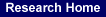reportThis report is an archived publication and may contain dated technical, contact, and link information
 Federal Highway Administration > Publications > Research > Infrastructure > Structures > Geosynthetic Reinforced Soil Integrated Bridge System Interim Implementation Guide
Publication Number: FHWA-HRT-11-026
Date: January 2011

# Geosynthetic Reinforced Soil Integrated Bridge System Interim Implementation Guide

## APPENDIX C. LRFD DESIGN PROCEDURE

### C.1 INTRODUCTION

An AASHTO LRFD procedure is presented in this appendix to permit the designer a choice of design methodology formats for GRS–IBS.( 9 ) This procedure is primarily a fit to the ASD solutionbut does include some LRFD features. For example, checking the external stability of earth–retaining structures is well defined by AASHTO LRFD. For internal stability of GRS structures, however, LRFD is not well defined yet. Since there is not enough statistical information available to fully calibrate LRFD for internal stability, the LRFD method has been fit to ASD. This means that the designer will arrive at the same answer for internal stability whether using ASD or LRFD. In the future, once more performance tests and case studies have been evaluated, the resistance factors for both vertical capacity and reinforcement strength may change from those presented in this appendix.

For LRFD, AASHTO defines the various load factors and load combinations that need to be considered in the design of bridge and transportation structures.(9) Table 16 and table 17 reproduce these load combinations and load factors. Resistance factors for external stability are also given by AASHTO.( 9 ) For direct sliding, a resistance factor for shear resistance ( Φr ) is included. For sliding of soil on soil, Φris equal to 1. For bearing capacity, the resistance factor ( Φbc ) is equal to 0.65. Finally, for global stability, the resistance factor is 0.65.

 Load Combination Limit State DC DD DW EH EV ES EL PS CR SH LL IM CE BR PL LS WA WS WL FR TU TG SE Use one of these at a time EQ IC CT CV Strength I (unless noted) γp 1.75 1.00 – – 1.00 0.50/1.20 γTG γSE – – – – Strength II γp 1.35 1.00 – – 1.00 0.50/1.20 γTG γSE – – – – Strength III γp – 1.00 1.40 – 1.00 0.50/1.20 γTG γSE – – – – Strength IV γp – 1.00 – – 1.00 0.50/1.20 – – – – – – Strength V γp 1.35 1.00 0.40 1.00 1.00 0.50/1.20 γTG γSE – – – – Extreme Event I γp γEQ 1.00 – – 1.00 – – – 1.00 – – – Extreme Event II γp 0.50 1.00 – – 1.00 – – – – 1.00 1.00 1.00 Service I 1.00 1.00 1.00 0.30 1.0 1.00 0.50/1.20 γTG γSE – – – – Service II 1.00 1.30 1.00 – – 1.00 0.50/1.20 – – – – – – Service III 1.00 0.80 1.00 – – 1.00 0.50/1.20 γTG γSE – – – – Service IV 1.00 – 1.00 0.70 – 1.00 0.50/1.20 – 1.0 – – – – Fatigue I–LL, IMand CEonly – 1.50 – – – – – – – – – – – Fatigue I II–LL, IMand CEonly – 0.75 – – – – – – – – – – –

Type of Load, Foundation Type, and
Method Used to Calculate Downdrag
Maximum Minimum

DC: Component and Attachments

DC: Strength IV only

1.25

1.50

0.90

0.90

DD: Downdrag

Piles, α Tomlinson Method

Piles, λ Method

Drilled shafts, O’Neill and Reese (1999) Method

1.40

1.05

1.25

0.25

0.30

0.35

DW: Wearing Surfaces and Utilities

1.50

0.65

EH: Horizontal Earth Pressure

• Active

• At–Rest

• AEP for anchored walls

1.50

1.35

1.35

0.90

0.90

N/A

EL: Locked–in Construction Stresses

1.00

1.00

EV: Vertical Earth Pressure

• Overall Stability

• Retaining Wall and Abutments

• Rigid Buried Structure

• Rigid Frames

• Flexible Buried Structures other than Metal Box Culverts

• Flexible Metal Box Culverts and Structural Plate Culverts with Deep Corrugations

1.00

1.35

1.30

1.35

1.95

1.50

N/A

1.00

0.90

0.90

0.90

0.90

ES: Earth Surcharge

1.50

0.75

### C.2 GRS–IBS DESIGN GUIDELINES

The following nine basic steps for the design of GRS–IBS are reproduced from section 4.4:

1. Establish project requirements.

2. Perform a site evaluation.

3. Evaluate project feasibility.

4. Determine layout of GRS–IBS.

6. Conduct external stability analysis.

7. Conduct internal stability analysis.

8. Implement design details.

9. Finalize GRS–IBS.

Once these steps are accomplished, the GRS–IBS can be constructed. The basic design guidelines are the same whether using ASD or LRFD. However, the detailed equations within step 6 and step 7 will differ between the two design methods. In this appendix, only the differences in step 6 and step 7 that result from conversion to the LRFD format are presented. Refer to section 4.4 section 4.3 for discussion on each of these design elements and the equivalent ASD equations and to section 4.5 section 4.4 for the ASD calculation.
AMENDED May 24, 2012

C.2.1 Step 6–Conduct an External Stability Analysis

The external stability of a GRS–IBS is evaluated by looking at the following potential external failure mechanisms:

• Direct sliding.

• Bearing capacity.

• Global stability.

C.2.1.1 Direct Sliding

The total factored driving force for LRFD (FR) is calculated in much the same way as in ASD (Fn)except load factors are applied to each component of thrust force. Equation 70 modifies equation 13 to include the load factors γEH MAX, γES MAX, and γLS, which are determined using table 16 and table 17.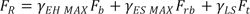(70)

The factored resisting force (RR) is calculated using equation 71. This equation is the LRFD modification of equation 14 that includes a shear resistance factor ( Φτ ). For sliding, Φτ is equal to 1.0. ( 9 )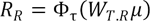(71)

Where Wt,R is determined using equation 72, which is equation 15 modified to include the appropriate load factors, ΦEV MIN, ΦDC MIN and ΦDC MIN, from table 17.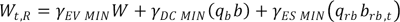(72)

For LRFD, the ratio of the factored resistance and the factored driving force must be greater than or equal to 1.0 (see equation 73). If not, consider lengthening the reinforcement at the base.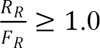(73)

C.2.1.2 Bearing Capacity

In this section, the ASD equations to evaluate bearing capacity have been modified to include the appropriate load and resistance factors of LRFD. Equation 74 is the LRFD version of equation 18.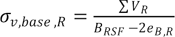(74)

Where ΣVR is the total factored vertical load on the GRS abutment (see equation 75), BRSF is the width of the RSF, and eB,R is the eccentricity of the resulting force at the base of the wall (see equation 76).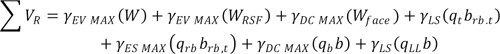(75)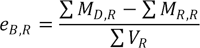(76)

Where γEV MAX, γLS, γES MAX and γDC MAX, are load factors found from table 16 and table 17, W is the weight of the GRS abutment, WRSF is the weight of the RSF, Wface is the weight of the facing elements, qtis the roadway LL, qrb is the road base DL, brb,t is the width of the traffic and road base surcharges over the GRS abutment, qb is the bridge DL, b is the width of the bridge seat, qLL is the bridge LL, ΣMD,R is the total factoreddriving moment, ΣMR,R is the total factored resisting moment, and ΣVR is the total factored verticalload. The moments should be calculated about the bottom center of the RSF length for the specific layout of the GRS abutment. If eB,Rn is negative, take eB,R equal to zero for the term BRSF–2eB,R.

The factored bearing capacity of the foundation (qR) can be found using equation 77. Based on AASHTO, the resistance factor ( Φbc) is equal to 0.65.( 9 )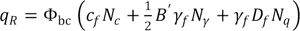(77)

Where cf is the cohesion of the foundation soil, Nc, Nγ, and Nq are dimensionless bearing capacitycoefficients (see table 4 ), Φfis the unit weight of the foundation soil, B' is the effective foundation width (equal to BRSF–2eB,R), and Df is the depth of the embedment.

The ratio of the factored bearing resistance and the factored applied pressure must be greater than or equal to 1.0 (see equation 78). If not, increase the width of the GRS abutment and RSF (by increasing the length of the reinforcement layers), replace the foundation soil with a more competent soil, or add embedment depth.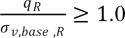(78)

C.2.1.3 Global Stability

According to AASHTO, the Service I Load Combination should be used to evaluate global stability.( 9 ) For the Service I limit state, the load factor is 1.0 for permanent loads. When the geotechnical parameters are not well defined or the slope does contain or support a structural element, the shear resistance factor is 0.65. This corresponds to a factor of safety of 1.5.

C.2.2 Step 7–Conduct Internal Stability Analysis

C.2.2.1 Vertical Capacity

It is recommended that the ultimate capacity be found empirically, if possible. A resistance factor for capacity ( Φcap) of 0.45 should be applied to the nominal vertical capacity (qn) to account for uncertainty. This resistance factor value is based on fitting to the ASD method.

C.2.2.1.1 Empirical Method: The factored applied pressure on the GRS abutment (Vapplied,f,emp) is equal to the sum of the vertical pressures on the bridge bearing area multiplied by their respective load factors (see equation 79). The vertical pressures include the bridge DL (qb) and LL (qLL).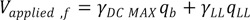(79)

Where γDC MAX and γLL are load factors found from table 16 and table 17. The factored applied pressure must be less than or equal to the factored vertical capacity (see equation 80). The resistance factor ( Φcap ) is equal to 0.45.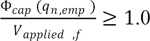(80)

C.2.2.1.2 Analytical Method: As an alternative, the load–carrying capacity of a GRS abutment can be evaluated using an analytical formula, referred to as the soil–geosynthetic composite capacity equation.( 11 )

The nominal ultimate load–carrying capacity (qn,an) of a GRS wall constructed with a granular backfillcan be determined by the soil–geosynthetic composite capacity equation, shown in equation 81.( 11 )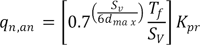(81)

Where Sv is the reinforcement spacing, dmax is the maximum grain size of the reinforced backfill, Tf is the ultimate strength of the reinforcement, and Kpr is the coefficient of passive earth pressure determined using equation 26.

The factored applied pressure on the GRS mass (Vapplied,f) is equal to the sum of the vertical forces multiplied by their respective load factors (see equation 82). This includes the bridge DL (qb) and LL (qLL). The DL due to the road base (qrb) and the LL due to the approach pavement (qt) are located behind the bearing area and are therefore not included in capacity calculations related to the bridge superstructure.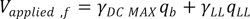(82)

Where γDC MAX and γLL are load factors found from table 16 and table 17. The factored applied pressure must be less than the factored ultimate capacity (see equation 83).The resistance factor ( Φcap ) is equal to 0.45.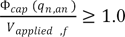(83)

C.2.2.2 Deformations

The method to estimate both vertical and lateral deformations is not dependent on the design code chosen (ASD or LRFD). Therefore, refer to section 4.4.7.2 to estimate deformations.

C.2.2.3 Required Reinforcement Strength

The factored required reinforcement strength in the direction perpendicular to the wall face ( Treq,f )can be determined analytically by equation 84. The required factored reinforcement strength should be calculated at each layer of reinforcement to ensure adequate strength throughout the GRS abutment. For the serviceability check (comparing the required strength to the reinforcement strength at 2 percent strain), the unfactored required reinforcement strength should be used (see equation 85).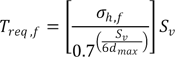(84)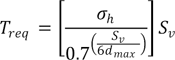(85)

Where Svis the reinforcement spacing, dmax is the maximum grain size of backfill, σh,fis the total factored lateral stress within the GRS abutment at a given depth and location (see equation 86), and σhis the total unfactored lateral stress within the GRS abutment at a given depth and location (equation 32).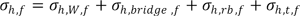(86)

Where σh,W,f is the factored lateral earth pressure using Rankine's active stress condition (see equation 87), σh,bridge,f is the factored lateral pressure due to the equivalent bridge load (see eqautaion 88), σh,rb,f is the factored lateral pressure due to the road base DL (see equation 89), and σh,t,f is the factored lateral pressure due to the roadway LL (see equation 90).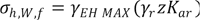(87)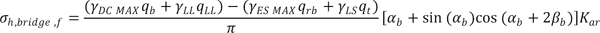(88)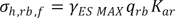(89)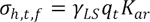(90)

Where γDC MAX, γES MAX, γLS, and γLL are load factors found from table 16 and table 17; qb, qrb, qt, and qLL are the bridge DL, road base DL, roadway LL and bridge LL, respectively; Kar is the active earth pressure coefficient for the reinforced fill; and αb and βbare the angles shown in table 15, found using equation 91 and equation 92, respectively.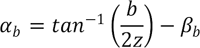(91)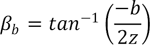(92)

For abutments, a minimum wide width tensile strength (Tf) of 4,800 lb/ft is required. A resistance factor for reinforcement strength ( Φreinf ) of 0.4 should be applied to the ultimate strength (Tf) to determine the factored reinforcement strength (Tf,f). In addition to a global reduction factor of 2.25 accounting for long-term strength losses (RFglobal) of the geosynthetic, a resistance factor for reinforcement strength (Φreinf) of 0.9 should be applied to the ultimate strength (Tf) to determine the factored reinforcement strength (Tf,f). The factored required reinforcement strength (Treq,f) must be less than this factored reinforcement strength (Tf,f), as shown in equation 93.
AMENDED May 24, 2012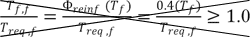(93)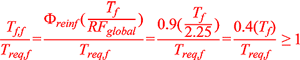(93)

AMENDED May 24, 2012

Since geosynthetic reinforcements of similar strength can have rather different load–deformation relationships depending on their material, it is important that the nominal (unfactored) Treq be less than the strength at 2 percent reinforcement strain. The strength of the reinforcement at 2 percent (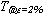) is often given by the geosynthetic manufacturer. If the unfactored Treqis greater than, either a different geosynthetic must be chosen or the ultimate strength must be increased.

### C.3 DESIGN EXAMPLE (LRFD): BOWMAN ROAD BRIDGE, DEFIANCE COUNTY, OH

In this section, the equations formatted for the LRFD method in section C.2 are demonstrated. For additional details and discussion in support of these calculations, see the corresponding sections of the design example in the ASD format contained in section 4.5 section 4.4.
AMENDED May 24, 2012

C.3.6 Step 6–Conduct an External Stability Analysis

C.3.6.1 Direct Sliding

The driving forces on the GRS abutment are comprised of the lateral forces due to the retained backfill, the road base and the traffic surcharge. The force due to the backfill is calculated in equation 94.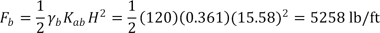(94)

The lateral force due to the road base and traffic surcharges are calculated in equation 95 and equation 96.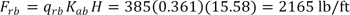(95)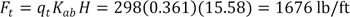(96)

The total factored driving force (FR) is then calculated in equation 97. The load factors are determined using table 16 and table 17.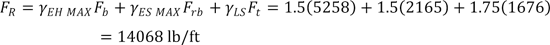(97)

The factored resisting force (RR) is calculated according to equation 71, where ΦT is the resistance factor for shear resistance (equal to 1.0 for sliding).( 9 ) The total resisting weight (Wt,R) includes the weight of GRS plus the weight of bridge beam plus the weight of the road base over the GRS abutment, as shown in equation 72. Since the LLs are not permanent, they cannot be counted as a resisting force. The total resisting weight is calculated in equation 98.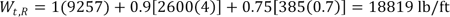(98)

The friction force (μ) is equal to tan Φcrit. The interface friction angle between the reinforced fill and the geotextile was measured at 39 degrees by conducting an interface direct shear test. The factored resisting force is calculated in equation 99.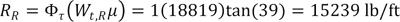(99)

This resisting force (15,239 lb/ft) is greater than the driving force (14,068 lb/ft); therefore, direct sliding is not an issue.

C.3.6.2 Bearing Capacity

Before calculating the factored applied vertical bearing pressure, the factored eccentricity of the resulting force at the base of the wall must be calculated.

The moments are calculated around the center of the base of the RSF. The driving moments (calculated as a counterclockwise moment) include the lateral force due to the retained backfill, the road base DL, and the roadway LL and are calculated in equation 100.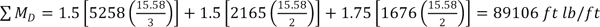(100)

The resisting moments (calculated as a clockwise moment) include the vertical force due to the bridge and road base DLs and the bridge and roadway LLs. The resisting moments are calculated in equation 101. The weight of the GRS abutment is also included as a resisting moment.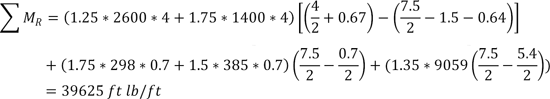(101)

The total vertical load is equal to the sum of the weight of the GRS abutment, the weight of the RSF, and the load due to the DLs (bridge and road base) and the LLs (bridge and roadway). The total vertical load is calculated in equation 102.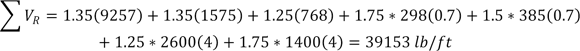(102)

The eccentricity of the resulting force at the base of the RSF is then calculated in equation 103.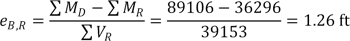(103)

The vertical pressure is a result of the weight of the GRS mass, the bridge seat load, and the traffic surcharge and is calculated in equation 104.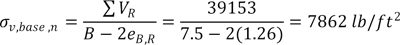(104)

The nominal bearing capacity is then calculated in equation 105. The degrees bearing capacity factors (Nc and Nγ) were found using table 4 for the foundation friction angle of 0.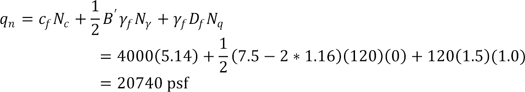(105)

Applying the resistance factor of 0.65 to the nominal bearing capacity, the factored bearing capacity (qR) is equal to 13,481 psf. The factored bearing capacity is greater than the factored vertical pressure (7,862 psf), so bearing capacity is not an issue in this case.

C.3.6.3 Global Stability

Global and compound stability was checked using the software program ReSSA. Global stability is not a problem.

C.3.7 Step 7–Conduct Internal Stability Analysis

C.3.7.1 Vertical Capacity

The ultimate capacity of a GRS abutment can be determined using two different methods: empirical or analytical.

C.3.7.1.1 Empirical Method: The empirical method uses the results of a performance test on a GRS composite material identical (or very similar) to that used in the field. The ultimate vertical capacity is found by looking at the applicable stress–strain curve from the performance test for a vertical strain of 5 percent (see figure 32 ). The capacity (qn,emp) is equal to 26 ksf. Note that the linear line extension shown in figure 32 is to project the capacity to 5 percent.

The factored applied pressure on the GRS mass (Vapplied,f) is found in equation 106: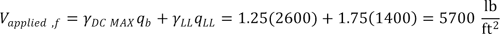(106)

The ratio of the factored vertical capacity (with a resistance factor of 0.45) to the factored applied pressure (Vapplied,f) must be greater than or equal to 1, as shown in equation 107.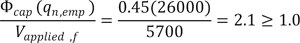(107)

C.3.7.1.2 Analytical Method: Alternatively, the nominal capacity is found analytically for a granular backfill, where Sv is equal to 8 inches, dmax is equal to 0.5 inches, Tf is equal to 4,800 lb/ft, and Φr is equal to 48 degrees. Note that although the spacing under the bridge bearing area is 4 inches, 8 inches was chosen to be conservative in the calculation for the entire mass.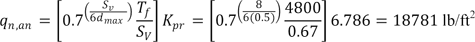(108)

Where the coefficient of passive earth pressure for the reinforced fill (Kpr) is found in equation 109.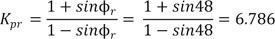(109)

The factored applied pressure on the GRS mass (Vapplied,f) is found in equation 110.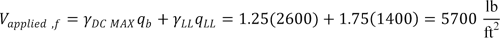(110)

The ratio of the factored ultimate capacity (with a resistance factor of 0.45) to the factored applied pressure (Vapplied,f) must be less than 1, as shown in equation 111.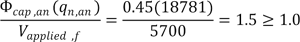(111)

C.3.7.2 Deformations

C.3.7.2.1 Vertical Deformation: The vertical strain is estimated by using figure 33 for the total bridge load (qb) of 2,600 lb/ft2. The vertical strain is, therefore, about 0.3 percent–under the tolerable limit of 0.5 percent. Note that the road base surcharge is not included because it does not act over the same location.

The vertical deformation is the product of the vertical strain and the height of the GRS mass and is calculated in equation 112.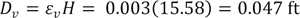(112)

C.3.7.2.2 Lateral Deformation: The lateral strain and deformation are found in equation 113 and equation 114.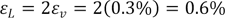(113)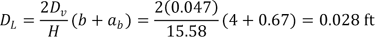(114)

C.3.7.3 Required Reinforcement Strength

The strength of the reinforcement used at Bowman Road Bridge is 4,800 lb/ft. Applying a resistance factor ( Φreinf ) of 0.4, the factored reinforcement strength (Tf,f) is 1,920 lb/ft Applying the resistance and global reduction factors of 0.9 and 2.25, respectively, the factored reinforcement strength (Tf,f) is 1,920 lb/ft. According to the manufacturer,is equal to 1,370 lb/ft. The maximum required reinforcement strength is found as a function of depth, as shown in equation 115.
AMENDED May 24, 2012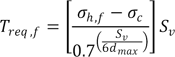(115)

The factored lateral stress ( σh,f ) is a combination of the factored lateral stresses due to the road baseDL ( σh,rb,f ), the roadway LL ( σh,t,f ), the GRS reinforced soil ( σh,W,f), and an equivalent bridgeload ( σh,bridge,f ). To simplify calculations, the roadway LL and road base DL can be extended across the abutment. The vertical components of these loads are then subtracted from the bridge DL and LL, giving an equivalent bridge load. The lateral stresses due to the equivalent bridge load are then calculated according to Boussinesq theory. The lateral stress is calculated for each depth of interest (each layer of reinforcement). All lateral stresses are calculated in table 18.

An example calculation for the required reinforcement strength at a depth (z) of 5.3 ft (the eighth reinforcement layer from the top) is shown in equation 116. First, the lateral pressure must be found. Remember, the location of interest is directly under the centerline of the bridge load (where x = 0.5b =0.5(4ft) = 2 ft).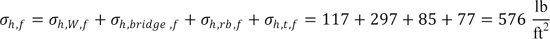(116)

Where the lateral pressure is found using equation 117 through equation 120.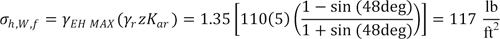(117)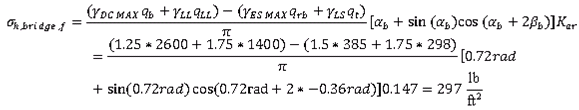(118)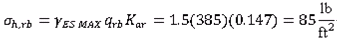(119)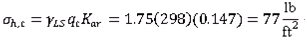(120)

The values for α and β are found in equation 121 and 122.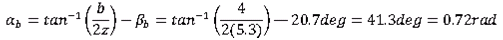(121)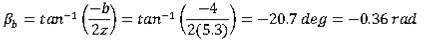(122)

The lateral earth pressure and required reinforcement strength should be found along the entire depth of the wall. The reinforcement spacing (Sv) for the required reinforcement strength calculation is 4 inches where secondary reinforcement layers are present and 8 inches where there are no secondary reinforcement layers. The depth at which secondary reinforcement layers are present is determined by applying the 8-inch reinforcement spacing for the entire height of the GRS mass. The depth at which the required reinforcement spacing does not exceed the factored reinforcement capacity of 1,920 lb/ft is the depth above which 4–inch spacing is required ( see table 18 ).

Table 18. Depth of bearing bed reinforcement calculations (LRFD).

z (ft) α β σh,bridge
(psf)
σh,rb
(psf)
σh,t
(psf)
σh,W
(psf)
σh,total,f
(psf)
σh,total
(psf)
Treq (lb/ft) Treq > Tf,f Treq (lb/ft) Treq >0.7 2.5 –1.2 669 85 77 15 845 592 1458 NO 1022 NO
1.3 2.0 –1.0 623 85 77 29 814 566 1406 NO 976 NO
2.0 1.6 –0.8 555 85 77 44 760 519 1312 NO 895 NO
2.7 1.3 –0.6 485 85 77 58 705 470 1217 NO 811 NO
3.3 1.1 –0.5 424 85 77 73 659 428 1136 NO 739 NO
4.0 0.9 –0.5 373 85 77 88 622 395 1074 NO 682 NO
4.7 0.8 –0.4 331 85 77 102 595 370 1027 NO 638 NO
5.3 0.7 –0.4 297 85 77 117 575 350 993 NO 605 NO
6.0 0.6 –0.3 268 85 77 131 562 336 969 NO 580 NO
6.7 0.6 –0.3 245 85 77 146 552 326 953 NO 563 NO
7.3 0.5 –0.3 225 85 77 160 547 319 944 NO 551 NO
8.0 0.5 –0.2 207 85 77 175 544 315 939 NO 543 NO
8.7 0.5 –0.2 192 85 77 190 544 312 939 NO 539 NO
9.3 0.4 –0.2 180 85 77 204 546 312 942 NO 538 NO
10.0 0.4 –0.2 168 85 77 219 549 312 947 NO 539 NO
10.7 0.4 –0.2 158 85 77 233 553 314 955 NO 542 NO
11.3 0.3 –0.2 149 85 77 248 559 317 965 NO 547 NO
12.0 0.3 –0.2 141 85 77 263 566 321 976 NO 554 NO
12.7 0.3 –0.2 134 85 77 277 573 325 989 NO 561 NO
13.3 0.3 –0.1 128 85 77 292 581 330 1003 NO 570 NO
14.0 0.3 –0.1 122 85 77 306 590 336 1018 NO 579 NO
14.7 0.3 –0.1 116 85 77 321 599 342 1034 NO 590 NO

Based on table 18, the required reinforcement strength does not exceed the allowable strength or the strength at 2 percent at any reinforcement layer. Therefore, no bearing bed reinforcement is needed. However, the minimum requirement is that the bearing bed reinforcement should extend through five courses of blocks. In actuality, six courses of block were chosen to extend the bearing reinforcement bed in this case (to a depth of 4 ft below the top of the wall). This was chosen to be conservative since this was the first bridge built with GRS technology. There should, therefore, be no issues with reinforcement strength in the abutment.

C.3.8 Step 8–Implement Design Details

All design details were considered. Since it is a skewed bridge, a bearing area of 3 ft was maintained along the length of the face wall. The bearing bed reinforcement schedule was also maintained across the abutment face due to the superelevation (see figure 35 ).

Federal Highway Administration | 1200 New Jersey Avenue, SE | Washington, DC 20590 | 202-366-4000
Turner-Fairbank Highway Research Center | 6300 Georgetown Pike | McLean, VA | 22101